# The Ultimate Beginner’s Guide to NumPy In Python

## NumPy basics with a lot of examplesPhoto by Boitumelo Phetla on Unsplash

Note: Most of the examples used to explain concepts of NumPy have been taken from Python For Data Analysis by Wes McKinney.

Let’s get started.

A ndarray is a generic multidimensional container for homogeneous data; that is, all of the elements must be the same type.

Every array has a shape, a tuple indicating the size of each dimension, and a dtype, an object describing the data type of the array:

`# randn returns elements from a standard normal distributiondata = np.random.randn(2,3)data`

Output:

`array([[-1.00945873, -0.14747028,  1.04654565],       [-0.69762101,  0.35370184, -0.08946465]])`

To check the type of each element we use:

`type(data.dtype)`

Output:

`numpy.dtype[float64]`

Arrays are important because they enable you to express batch operations on data without writing any for loops. NumPy users call this vectorization. Any arithmetic operations between equal-size arrays apply the operation element-wise: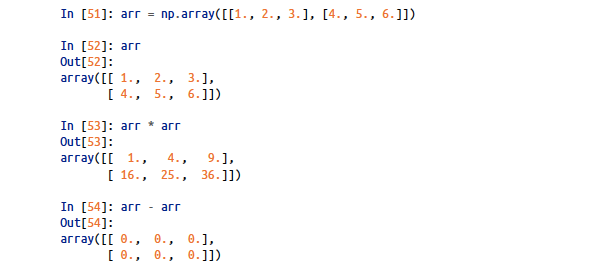Basic Arithmetic operations with NumPy (screenshot from the book mentioned above)
`# arithmetic operations with a scalar will be applied to each and every elementmy_arr1 = my_arr * 2print(my_arr1)`

Output:

`[[ 2  4  6] [ 8 10 12]]`

NumPy array indexing is a rich topic, as there are many ways you may want to select a subset of your data or individual elements.

In 1-dimensional arrays, we can simply index it using start:end where the start index element is included but the end index element is excluded.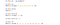Indexing in a 1-dimensional numpy array

We also took 5:8 as updated it to 12. We can then see that updating the slice value updated the original array as well.

To update all the values in the array we can use arr[:] = 12. It will update the whole array to 12 starting from the 1st index to its (length-1).

With 2-dimensional arrays, elements at each index are not scalars but rather 1-dimensional arrays.

It stays the same with higher dimensions as well. In multidimensional arrays, if you omit later indices, the returned object will be a lower-dimensional ndarray consisting of all the data along the higher dimensions.

`arr2d = np.array([[1, 2, 3], [4, 5, 6], [7, 8, 9]])arr2dOutput: array([7, 8, 9])`

Thus, individual elements can be accessed recursively. But that is a bit too much work, so you can pass a comma-separated list of indices to select individual elements.

So these are equivalent:

`arr2dOutput: 3arr2d[0, 2]Output: 3`

We can even do indexing with slices. Let us understand it with an example.

Here it will pick up elements that are 1d arrays itself at positions 0 and 1 which are [1,2,3] and [4,5,6] respectively.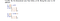Indexing with slices

We can even slice both rows and column elements together as:

`arr2d[:2, 1:]Output: array([[2, 3],        [5, 6]])`

Universal Functions or UFuncs perform element-wise operations on our ndarrays. We have unary or binary ufuncs.

`my_arr = np.arange(20)my_arr = my_arr.reshape((5,4))my_arr`

Output:

`array([[ 0,  1,  2,  3],       [ 4,  5,  6,  7],       [ 8,  9, 10, 11],       [12, 13, 14, 15],       [16, 17, 18, 19]])`

On applying a unary ufunc like np.sqrt(my_arr) we get:

`array([[0.        , 1.        , 1.41421356, 1.73205081],       [2.        , 2.23606798, 2.44948974, 2.64575131],       [2.82842712, 3.        , 3.16227766, 3.31662479],       [3.46410162, 3.60555128, 3.74165739, 3.87298335],       [4.        , 4.12310563, 4.24264069, 4.35889894]])`

We also have binary ufuncs which takes 2 arrays as input:

`x = np.random.randn(10)y = np.random.randn(10)print(x)print("\n")print(y)`

Output:

`[ 0.4803378   1.43452441  0.56222455  0.4097964  -0.28604575  0.83715151  0.02814258  0.51104714 -0.21852359  1.57191921][-0.87110468  0.31741718 -0.64925443 -0.76802201  0.30300398  2.43681536  0.4366532   0.42144164 -1.49904037 -0.08998904]`

On applying a binary ufunc like np.maximum(x,y) it will compare all the elements of x and y array with each other and return the maximum element:

`array([ 0.4803378 ,  1.43452441,  0.56222455,  0.4097964 ,  0.30300398,       2.43681536,  0.4366532 ,  0.51104714, -0.21852359,  1.57191921])`

There is a long list of universal functions available in NumPy that makes our life easier. Pick and try a few of them.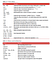A long list of Universal Functions (screenshot from the book mentioned above)

Using NumPy arrays to perform array-oriented operations is highly powerful as compared to using python loops or list comprehension.

We can perform a complex computation of sqrt(x² + y²) across a regular grid of values with just a few lines of code.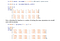A complex computation of sqrt(x² + y²) across a regular grid of values

Even performing operations based on some conditional logic can be performed easily with NumPy arrays.

`xarr = np.array([1.1, 1.2, 1.3, 1.4, 1.5])yarr = np.array([2.1, 2.2, 2.3, 2.4, 2.5])cond = np.array([True, False, True, True, False])`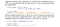Expressing Conditional Logic as Array Operations

I tried it with a million data points and was surprised to see that NumPy array operations were able to perform around 100 times faster than the python list comprehension.

We can even perform statistical operations with ndarrays.

`my_arr = np.random.randn(4,4)my_arr`

Output:

`array([[ 0.6052009 ,  0.11951734, -0.80470578, -2.54784742],       [ 0.5399688 ,  1.66262227,  0.81955271, -0.55774819],       [ 0.41959478,  0.2194956 , -1.81219585,  0.85218674],       [ 1.6213405 ,  0.0761287 , -0.32877757,  1.07090786]])`

Now before trying out sum, mean, std, etc. operations we need to understand the concept of axis here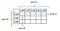Picture explaining the concept of axis in ndarrays

np.sum(my_arr): 1.9552414143838845

np.mean(my_arr, axis=1): array([-0.65695874, 0.6160989 , -0.08022968, 0.60989987])

We also have cumsum, cumprod, etc. operations to calculate the cumulative sum or product of a stretched array or along an axis.

`arr = np.arange(1,15, 2)arr`

Output: array([ 1, 3, 5, 7, 9, 11, 13])

`np.cumsum(arr)`

Output: array([ 1, 4, 9, 16, 25, 36, 49])

Pick and try a few of them from the table below.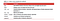Basic statistical methods in numpy arrays

NumPy arrays can be sorted in-place with the sort method.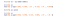Sorting in numpy arrays

Now while sorting in higher dimensional NumPy arrays we can even mention the axis along which we need to sort.

`arr = np.random.randn(5, 3)arr`

Output:

`array([[ 0.1357564 , -1.21689356, -0.03179582],       [-0.018589  ,  0.76169735, -0.09404734],       [-0.27728709, -0.27615453, -0.80852859],       [ 0.08735213,  0.37884326,  2.23298412],       [-2.33812985, -1.53835618, -0.92607446]])`

After applying arr.sort(axis=1) we get:

sorted output along axis 1:

`array([[-1.21689356, -0.03179582,  0.1357564 ],       [-0.09404734, -0.018589  ,  0.76169735],       [-0.80852859, -0.27728709, -0.27615453],       [ 0.08735213,  0.37884326,  2.23298412],       [-2.33812985, -1.53835618, -0.92607446]])`

We even have a kind parameter to our sort method which can take up any value from {‘quicksort’, ‘mergesort’, ‘heapsort’, ‘stable’}. The default is ‘quicksort’.

NumPy has some basic set operations for one-dimensional ndarrays. A commonly used one is np.unique, which returns the sorted unique values in an array

`names = np.array(['Bob', 'Joe', 'Will', 'Bob', 'Will', 'Joe', 'Joe'])names`

Output:

`array(['Bob', 'Joe', 'Will', 'Bob', 'Will', 'Joe', 'Joe'], dtype='<U4')`

On applying np.unique(names) we get:

`array(['Bob', 'Joe', 'Will'], dtype='<U4')`

Similarly, we can apply union1d and it will compute the sorted union of elements:

`np.union1d(names, ["Hie"])`

Output:

`array(['Bob', 'Hie', 'Joe', 'Will'], dtype='<U4')`

Again I will say the same thing. Pick and try a few of them from the table below.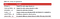List of Array set of operations

Note: NumPy is able to save and load data to and from disk either in text or binary format using load, save, savez, etc. methods. But we will not be discussing it over here as pandas or other tools are more preferred than this.

I have left out few advanced concepts on purpose as this article was meant to be a beginner’s guide. I will be discussing them in another article.

Let me know if you face any difficulties in the comments.

## Analytics Vidhya

Analytics Vidhya is a community of Analytics and Data…

## Analytics Vidhya

Analytics Vidhya is a community of Analytics and Data Science professionals. We are building the next-gen data science ecosystem https://www.analyticsvidhya.com

Written by

## Rahul Kapoor

Implementation Analyst || Learning Analytics and Machine Learning## Analytics Vidhya

Analytics Vidhya is a community of Analytics and Data Science professionals. We are building the next-gen data science ecosystem https://www.analyticsvidhya.com

## More From Medium

Medium is an open platform where 170 million readers come to find insightful and dynamic thinking. Here, expert and undiscovered voices alike dive into the heart of any topic and bring new ideas to the surface. Learn more

Follow the writers, publications, and topics that matter to you, and you’ll see them on your homepage and in your inbox. Explore

If you have a story to tell, knowledge to share, or a perspective to offer — welcome home. It’s easy and free to post your thinking on any topic. Write on Medium